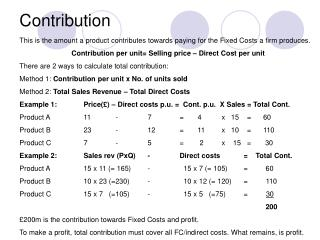DownloadDownload PresentationContribution

# Contribution

Download Presentation## Contribution

- - - - - - - - - - - - - - - - - - - - - - - - - - - E N D - - - - - - - - - - - - - - - - - - - - - - - - - - -
##### Presentation Transcript

1. Contribution This is the amount a product contributes towards paying for the Fixed Costs a firm produces. Contribution per unit= Selling price – Direct Cost per unit There are 2 ways to calculate total contribution: Method 1: Contribution per unit x No. of units sold Method 2: Total Sales Revenue – Total Direct Costs Example 1: Price(£) – Direct costs p.u. = Cont. p.u. X Sales = Total Cont. Product A 11 - 7 = 4 x 15 = 60 Product B 23 - 12 = 11 x 10 = 110 Product C 7 - 5 = 2 x 15 = 30 Example 2: Sales rev (PxQ) - Direct costs = Total Cont. Product A 15 x 11 (= 165) - 15 x 7 (= 105) = 60 Product B 10 x 23 (=230) - 10 x 12 (= 120) = 110 Product C 15 x 7 (=105) - 15 x 5 (=75) = 30 200 £200m is the contribution towards Fixed Costs and profit. To make a profit, total contribution must cover all FC/indirect costs. What remains, is profit.